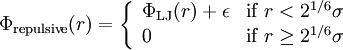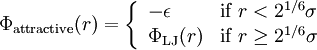Weeks-Chandler-Andersen perturbation theory

(diff) ← Older revision | Latest revision (diff) | Newer revision → (diff)

The Weeks-Chandler-Andersen perturbation theory  is based on the following decomposition of the intermolecular pair potential (in particular, the Lennard-Jones potential):

The reference system pair potential is given by (Eq, 4):$\Phi_{\rm repulsive} (r) = \left\{ \begin{array}{ll} \Phi_{\rm LJ}(r) + \epsilon & {\rm if} \; r < 2^{1/6}\sigma \\ 0 & {\rm if} \; r \ge 2^{1/6}\sigma \end{array} \right.$

and the perturbation potential is given by (Eq, 5 Ref. 1):$\Phi_{\rm attractive} (r) = \left\{ \begin{array}{ll} -\epsilon & {\rm if} \; r < 2^{1/6}\sigma \\ \Phi_{\rm LJ}(r) & {\rm if} \; r \ge 2^{1/6}\sigma \end{array} \right.$